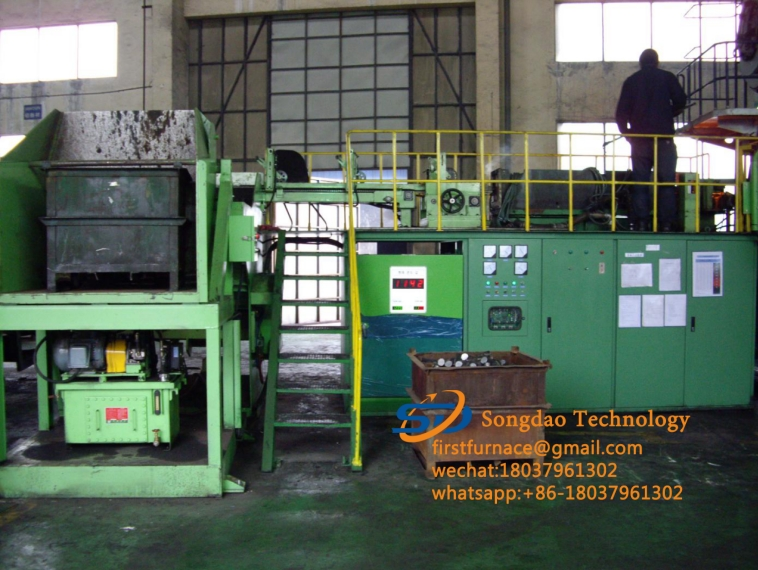### How to calculate the power of round steel and bar material using induction heating furnace?

P=（0.168×1050℃×60kg）/（0.24×0.65×60秒）=1130KW

How to calculate the power of round steel and bar material using induction heating furnace?

How to calculate the power required to heat round steel with a diameter of 40 and a length of 6 meters in an intermediate frequency induction heating furnace? Heating temperature is 1050℃

Heating time is 60 seconds.

1kg of steel is heated from 25 degrees to 1250 degrees in 60 seconds. Assuming that the efficiency of the heater is 0.5, the required power is: 0.168×<1250-25>×1÷0.24÷0.5÷60=23.8 kilowatts.

Calculate the mass of round steel with a diameter of 40 and a length of 6 meters as 60kg

P=(0.168×1050℃×60kg)/(0.24×0.65×60 seconds)=1130KW